Courses

# Unit & Dimensions Vectors(Part- 1) - Physics, Solution by DC Pandey NEET Notes | EduRev

## DC Pandey (Questions & Solutions) of Physics: NEET

Created by: Ciel Knowledge

## NEET : Unit & Dimensions Vectors(Part- 1) - Physics, Solution by DC Pandey NEET Notes | EduRev

The document Unit & Dimensions Vectors(Part- 1) - Physics, Solution by DC Pandey NEET Notes | EduRev is a part of the NEET Course DC Pandey (Questions & Solutions) of Physics: NEET.
All you need of NEET at this link: NEET

Section-I
Single Correct Option
Ques 1: Which one of the following has the dimensions of pressure?
(a) [ML-2T-2
(b) [M-1 L-1
(c) [MLT-2
(d) [ML-1T-2]
Ans: [ML-1T-2]
Sol: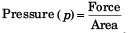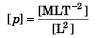= [ML-1T-2]
Option (d) is correct.

Ques 2: Which of the following will have the dimensions of time
(a) LC
(b) R/L
(c) L/R
(d) C/L
Ans: L/R
Sol:
W = I2 Rt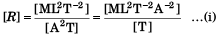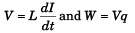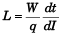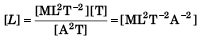Using Eq. (i)
[R] = [L]/[T]
i.e., [T] = [L/R]
Option (c) is correct.

Ques 3: The force F on a sphere of radius a moving in a medium with velocity v is given by F = 6πηa v. The dimensions of-η are
(a) [ML-3
(b) [MLT-2
(c) [MT- 1
(d) [ML-1T-1]
Ans: [ML-1T-1]
Sol:

F= 6π ηav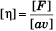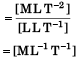Option (d) is correct.

Ques 4: The dimensional formula for magnetic flux is
(a) [ML2T -2A-1
(b) [ML3T-2A-2]
(c) [M0 L-2T-2A-2
(d) [ML2T-1A2]
Ans: [ML2T -2A-1]
Sol:
φ = Li
∴  [φ] = [L] [i]
=[ML2 T-2 A-2] [A]
=[ML2 T-2 A-1]
Option (a) is correct.

Ques 5: Dimensions of linear impulse are
(a) [ML-2T-3]
(b) [ML-2]
(c) [MLT -1]
(d) [MLT-2]
Ans: [MLT -1]
Sol:
Linear impulse (I) = F × Δt
[I ] = [MLT-2] [T]
= [MLT-1]
Option (c) is correct.

Ques 6: What is the dimensional formula of gravitational constant?
(a) [ML2T-2
(b) [ML-1T -1
(c) [M-1L3 T-2
(d) None of these
Ans: [M-1L3 T-2]
Sol: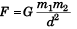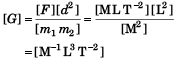Option (c) is correct.

Ques 7: Using mass (M), length (L), time (T) and current (A) as fundamental quantities, the dimension of permeability is
(a) [M-1LT-2A]
(b) [ML-2T-2A-1
(c) [MLT -2A-2
(d) [MLT -1 A-1]
Ans: [MLT -2A-2]
Sol: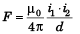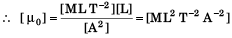Option (c) is correct.

Ques 8: The equation of a wave is given by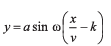where ω is angular velocity and v is the linear velocity. The dimensions of k will be
(a) [T2
(b) [T-1
(c) [T]
(d) [LT]
Ans: [T]
Sol: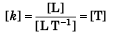Option (c) is correct.

Ques 9: A force is given by F = at + bt2
where t is the time. The dimensions of a and b are
(a) [MLT -4] and [MLT 1
(b) [MLT-1] and [MLT0
(c) [MLT-3] and [MLT -4
(d) [MLT -3] and [MLT0]
Ans: [MLT-3] and [MLT -4]
Sol: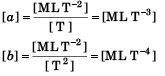Option (c) is correct.

Ques 10: The dimensional formula for Planck’s constant and angular momentum are
(a) [ML2T-2] and [MLT-1
(b) [ML2T-1] and [ML2T-1
(c) [ML3T1] and [ML2T -2
(d) [MLT-1] and [MLT-2]
Ans: [ML2T-1] and [ML2T-1]
Sol: ∴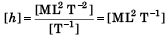Angular momentum (J) = nh/2π
[J] = [h] = [ML2 T-1]
Option (b) is correct.

Ques 11: If the energy (E), velocity (v) and force (F) be taken as fundamental quantities, then the dimensions of mass will be
(a) Fv-2 (b) Fv-1 (c) Ev-2 (d) Ev2
Ans: Ev-2
Sol: [Energy] = [ML2T-2]
= -[M] [LT-1]2
∴ [Mass] = [Ev-2]
Option (c) is correct.

Ques 12: The dimension of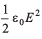(ε0 is the permittivity of free space and E is electric field), is
(a) [ML2T-1
(b) [ML- 1T-2
(c) [ML2T-2
(d) [MLT-1]
Ans: [ML- 1T-2]
Sol: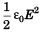= Energy density = Energy/Volume
∴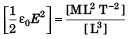= [ML-1 T-2]
Option (b) is correct.

Ques 13: The dimensions of a/b in the equation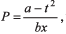where P is pressure, x is distance and t is time, are
(a) [M2 LT -3
(b) [MT-2
(c) [LT-3
(d) [ML3 T-1]
Ans: [MT-2]
Sol: [a] = [T2]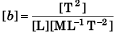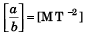Option (b) is correct.

Ques 14: Dimension of velocity gradient is
(a) [M°L°T-1
(b) [ML- 1T-1
(c) [M°LT-1
(d) [ML°T-1]
Ans: [M°L°T-1]
[Velocity gradient]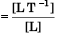= [T-1]
= [M0 L0 T-1]
Option (a) is correct.

Ques 15: If force F, length Land time T are taken as fundamental units, the dimensional formula for mass will be
(a) [FL- 1T2
(b) [FLT-2
(c) [FL- 1T-1
(d) [FL5T2]
Ans: [FL- 1T2]
Sol: [Force] = [MLT-2]

Ques 16: Which of the following is the dimension of the coefficient of friction? (a) [M2 L2T]
(b) [M° L° T°]
(c) [ML2T-2
(d) [M2 L2T-2]
Ans: [M° L° T°]
Sol: Coefficient of friction (μ)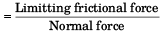∴   [μ] = [M0 L0 T0]
Option (b) is correct.

Ques 17: If C and R denote capacitance and resistance, then dimensions of CR will be
(a) [M°L°TA°]
(b) [ML°TA-2
(c) [ML°TA2
(d) [ML°T2A-2]
Ans: [M°L°TA°]
Sol: q = CV
and V = iR
∴ q = iCR
it = iCR
⇒ [CR] = [t] = [M0 L0 T A0]
Option (a) is correct.

Ques 18: The unit of permittivity of free space, ε0 is
(a) coulomb/newton-metre

(b) newton-metre2/coulomb2
(c) coulomb2/newton-metre2
(d) coulomb2 /(newton -metre)2
Ans: newton-metre2/coulomb2

Sol: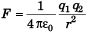∴ Unit of ε0 = Newton-metre2 /coulomb2.
Option (b) is correct.

Ques 19: The ratio of the dimensions of Planck’s constant and that of the moment of inertia is the dimension of
(a) frequency
(b) velocity
(c) angular momentum
(d) time
Ans: frequency
Sol: Angular momentum (J) = nh/2π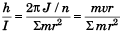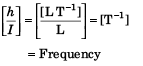Option (a) is correct.

Ques 20: The velocity v of a particle at time t is given by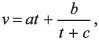where a, b and c are constants. The dimensions of a, b and c are respectively
(a) [LT-2], [L] and [T]
(b) [L2], [T] and [LT2]
(c) [LT2], [LT] and [L]
(d) [L], [LT] and [T2]

Ans: [LT-2], [L] and [T]
Sol: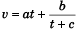[c] = [T]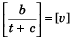or [b] = [LT-1] [T] = [L]
[at] = [v] = [LT-1]
⇒ [a] = [LT-2]
Option (a) is correct.

Ques 21: Given that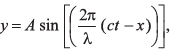where y and x are measured in metres. Which of the following statements is true ?
(a) The unit of λ is same as that of x and A
(b) The unit of λ, is same as that of x but not of A
(c) The unit of c is same as that of 2π/λ
(d) The unit of (ct - x) is same as that of 2π/λ

Ans: The unit of λ is same as that of x and A
Sol: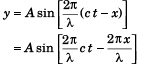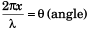[x] = [λ] = [L]
Further, y = A sinθ
∴ [A] = [y] = [L]
Option (a) is correct.

Ques 22: The physical quantity having the dimensions [M-1L-3T3A2] is
(a) resistance
(b) resistivity
(c) electrical conductivity
(d) electromotive force
Sol: [X] = [M-1 L-3 T3 A2]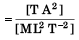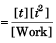∴ X is resistance.  [∴W = i2 Rt]

Ques 23: The torque of force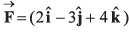newton acting at the point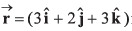metre about origin is (in N-m)
(a)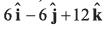(b)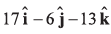(c)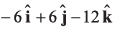(d)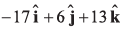Sol: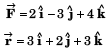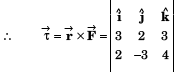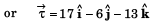Ques 24: If a unit vector is represented by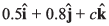the value of c is
(a) 1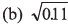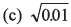(d) 0.39

Ans: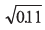Sol: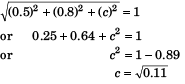Option (b) is correct.

Ques 25: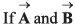are two vectors such that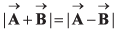he angle between vectors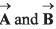is
Sol: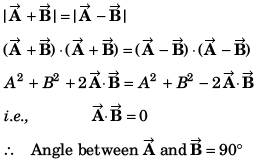Ques 26: The vector sum of two forces is perpendicular to their vector differences. In that case, the forces
(a) are not equal to each other in magnitude
(b) cannot be predicted
(c) are equal to each other
(d) are equal to each other in magnitude
Ans: are equal to each other in magnitude
Sol: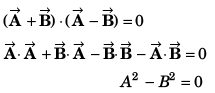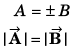Option (d) is correct.

Ques 27: Which one of the following is a scalar quantity?
(a) Displacement
(b) Electric field
(c) Acceleration
(d) Work
Ans: Work
Sol: Work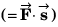is a scalar quantity.
Option (d) is correct.

Ques 28: Which one of the following is not the vector quantity?
(a) Torque
(b) Displacement
(c) Velocity
(d) Speed
Ans: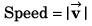Option (d) is correct.

Ques 29: What is the dot product of two vectors of magnitude 3 and 5, if angle between them is 60°?
(a) 5.2
(b) 7.5
(c) 8.4
(d) 8.6
Ans: 7.5
Sol: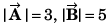and angle between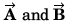is 60°.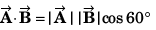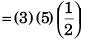= 7.5
Option (b) is correct.

Ques 30: Two vectors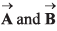are such that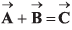and A2 + B2 = C2.
If 0 is the angle between positive direction ofthen the correct statement is
(a) θ = π
(b) θ = 2π/3
(c) θ = 0
(d) θ = π/2
Ans: θ = π/2
Sol: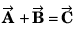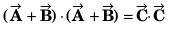or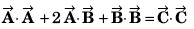or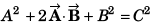or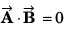or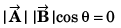or cos θ = 0
or θ = π/2
Option (d) is correct.

Offer running on EduRev: Apply code STAYHOME200 to get INR 200 off on our premium plan EduRev Infinity!

210 docs

,

,

,

,

,

,

,

,

,

,

,

,

,

,

,

,

,

,

,

,

,

,

,

,

;